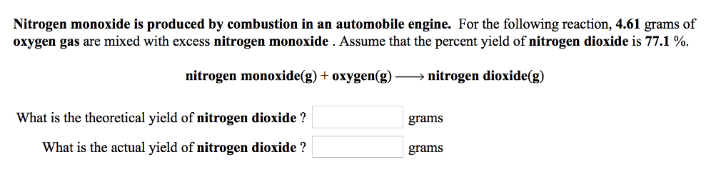# Problem: Nitrogen monoxide is produced by combustion in an automobile engine. For the following reaction, 4.61 grams of oxygen gas are mixed with excess nitrogen monoxide. Assume that the percent yield of nitrogen dioxide is 77.1 %. nitrogen monoxide (g) + oxygen (g) → nitrogen dioxide (g) What is the theoretical yield of nitrogen dioxide ? What is the actual yield of nitrogen dioxide ?

###### FREE Expert Solution
90% (172 ratings)###### Problem Details

Nitrogen monoxide is produced by combustion in an automobile engine. For the following reaction, 4.61 grams of oxygen gas are mixed with excess nitrogen monoxide. Assume that the percent yield of nitrogen dioxide is 77.1 %.

nitrogen monoxide (g) + oxygen (g) → nitrogen dioxide (g)

What is the theoretical yield of nitrogen dioxide ?

What is the actual yield of nitrogen dioxide ?